## AP 10th Maths Answer Key 2023

AP 10th Pre final Answer Key 2023 AP SSC Maths key Sheets PDF Download 2023 . Key sheets for the 2023-2024 pre-public Examination. AP Maths 10th Class Pre-Final Answer Keys 2023: Candidates who took the pre-final examinations can review the question paper and answer key. AP SSC Pre Final Exam Maths Answer key Download 2023 Principles of Evaluation, Official key sheet download.

1Q: LCM of 12, 15 and 21 is 420.

12 = (2 × 2 × 3) = 22 × 31,

15 = (3 × 5) = 31 × 51, and

21 = (3 × 7) = 31 × 71

LCM (12, 15, 21) = 420

2: Write the roster form of the set {x:x is a natural number less than 6}.

Ans:

Natural numbers less than 6 are: 1,2,3,4,5.

This can be written as:

{1,2,3,5}

3: The maximum number of zeroes which a quadratic polynomial can have is 2

The degree of a quadratic polynomial is 2.

5:  Find the roots of equation 5x26x2=0

5x2 – 6x – 2 = 0 ……………………………. (1)

On multiplying (1) by 5, we get 25x2 – 30x -10 = 0

(5x)2 – 2(5x)(3) +9 – 9 – 10 = 0

(5x3)2 = 19

Taking square root on both sides, we get

5x – 3 = ±19

5x = ±19

5x = 3 + 19 5x = 3 – 19

x = (3 + 19)/5 x = (3 – 19)/5

Therefore (3 + 19)/5 and (3 – 19)/5 are the roots of the given quadratic equation.

6: State Thale’s theorem

Thales theorem states that:

If a line is drawn parallel to one side of a triangle, then it divides the other two sides in the same ratio.
This theorem is also called as basic proportionality theorem.
7: The number of tangents drawn at the end points of the diameter is 2.
8: The side of a cube is 4 cm long. Find its volume.

The correct option is D 64cm3
Given: Length of each side = 4 cm
Volume of a cube = Side3
(4cm)3
64cm3
Therefore, volume of the given cube is 64cm3.

10: “You are observing top of your school building at an angle of elevation 60° from a point which is at 20 meters distance from foot of the building”.
Draw a rough diagram to the above situation.

Solution

Let,

BC=h be the height of the building,

The angle of elevation to the top of school is 60

and

20 mtr is the distance from the foot of the building.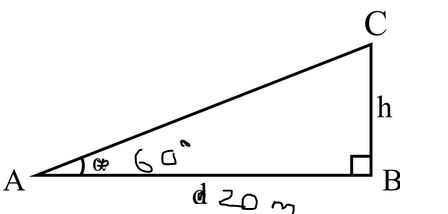11: If P(E) = 0.05, then the probability of ‘not E’ is .

The correct option is 0.95
P(E) = 0.05
Since P(E) + P (not E) = 1
P (not E) = 1 – P(E) = 1 – 0.05 = 0.95

12: Find the mean of the given data. 2, 3, 7, 6, 6, 3, 8

The sum of the data is: 2 + 3 + 7 + 8 + 11 + 6 + 5 = 42

The number of data points is 7

The mean is 42 divided by 7, which is equal to 6.

So, the mean of the data 2, 3, 7, 8, 11, 6, and 5 is 6.

13: If A = {3, 4, 5, 6} B = {5, 6, 7, 8, 9} then illustrate A ∩ B in Venn diagram.

14: 6 pencils and 4 pens together cost 50 whereas 5 pencils and 6 pens together cost ₹ 46. Express the above statements in the form of Linear equations.

15: Check whether (x – 2) 2 + 1 = 2x – 3 is a quadratic equation or not.

It is of the form .
Therefore, the given equation is a quadratic equation.
16: Write the formula to find nth term of A.P. and explain the terms in it.

Assume that a1, a2, a3,… be an arithmetic progression (AP), in which first term ais equal to “a” and the common difference is taken as “d”, then the second term, third term, etc can be calculated as follows:

Second term, a2 = a+d

Third term, a3 = (a+d)+d = a+2d,

Fourth term, a4 = (a+2d)+d = a+3d, and so on.

Therefore, the nth term of an AP (an) with the first term “a” and common difference “d” is given by the formula:

nth term of an AP, an = a+(n-1)d.

(Note: The nth term of an AP (an) is sometimes called the general term of an AP, and also the last term in a sequence is sometimes denoted by “l”.)

17: Find the distance between the two points (7, 8) and (-2, 3).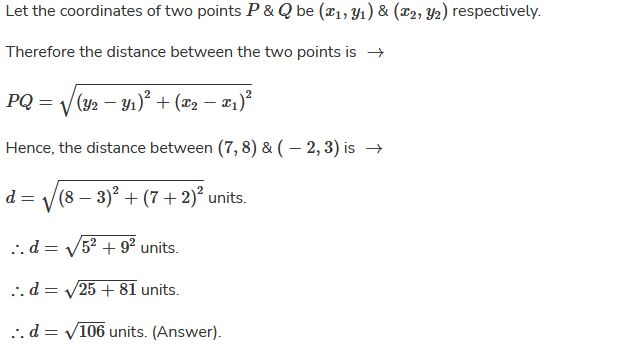18: From a point Q, the length of the tangent to a circle is 24 cm, and the distance of Q from the centre is 25 cm. Find the radius of the circle.

## Let QP be the tangent, such that, Point of contact is P.

Length of the tangent to a circle = 24cm
$$PQ=24cm$$
Let O be the centre of the circle.
We have to find the radius
Since QP is tangent
perpendicular to     (Since, Tangent is Perpendicular to Radius at the point of contact)
So,
So apply Pythagoras theorem to right triangle, ;
19 If cosA = 12 / 13 then find sinA and tanA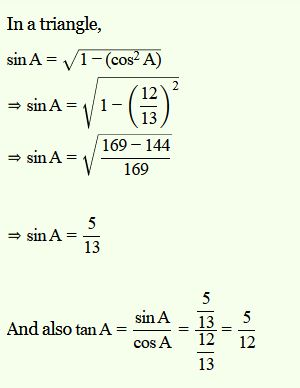20 A die is thrown once. Find the probability of getting(i) a prime number;
(ii) an odd numberWe use the basic formula of probability to solve the problem.Number of outcomes on throwing a die is (1, 2, 3, 4, 5, 6) = 6Number of prime numbers on dice are 1, 3 and 5 = 3(i) Probability of getting a prime number = Number of prime numbers/total number of outcomes= 3/6 = 1/2

(ii) Total number of odd numbers are 1, 3 and 5 = 3

Probability of getting a odd number = Number of odd numbers/total number of outcomes

= 3/6 = 1/2

21: If 2 log 5 + 1/2 log 9 – log 3 = log x then find the value of x

The given equation isWe need to find the value of x.

Using power property of logarithm, the given equation can be rewritten asOn comparing both sides we get22

If  and  are two zeroes of the polynomial find all the zeroes of the given polynomial.

## Let

Given : 3 and -3 are the zeroes of the polynomial  and  are factors of  and consequently  is factor of
Divide  by  we getPut

or  or  or
hence all the zeros of the given polynomial are  and

23 Solve the following system by elimination method:

## Given equations are

….(1)
and  ….(2)
Multiply equation (1) by  and equation (2) by , we get
….(3)
and  …. (4)
Subtract both the equations to eliminate , we get
Substitute this value in equation (1),
Therefore, the solution is .
24  Rohan’s mother is 26 years older than him. The product of their ages after 3 years will be 360 years. Write the quadratic equation to find Rohan’s present age.

Let take Rohan’s age = x years
Hence, his mother’s age = x+26
3 years from now
Rohan’s age = x+3
Age of Rohan’s mother will =x+26+3=x+29
The product of their ages 3 years from now will be 360 so that
(x+3)(x+29)=360
x2+29x+3x+87=360
x2+32x+87360=0
x2+32x273=0
x2+39x7x273=0
x(x+39)7(x+39)=0
(x+39)(x7)=0
Either x+39=0orx7=0
x=39 or x=7
As, age cannot be in a negative value
hence, x=7,Rohan’s age = 7 years
his mother’s age = 7+26=33 years

25 Draw a tangent to a given circle with centre ‘O’ from a point ‘R’ outside the circle. How many tangents can be drawn to the circle from that point?

Let O be center of circle and R be a point outside it.

Draw tangent to circle at A and B through R

Only two tangents can be drawn from a given point outside the circle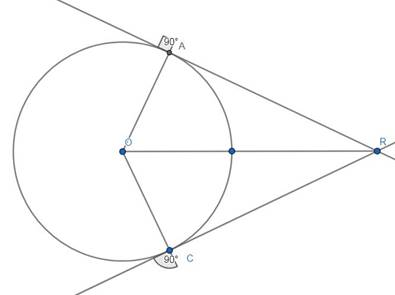26 An oil drum is in the shape of a cylinder having the following dimensions:
Diameter is 2 m and height is 7 meters. The painter charges ₹ 3 per m² to paint the drum. Find the total charges to be paid to the painter for 10 drums.

Given,

Diameter of an oil drum(cylinder) = 2m

Height of the oil drum = 7m

The painter charges 5 per meter to paint the drum.

To find out,

Find the total charges to be paid to the painter for 10 drums.

Solution:

Radius of the oil drum is 1 meter.

Total surface area of a cylindrical drum = 2×π×r(r + h) = 2×22/7×1(1 + 7) = 2 ×22/7 × 8

= 352/7 = 50.28 m^2

Therefore the total surface area of cylinder is 50.28 meter square.

Painting charge per 1 m² is rupees 5.

Charge per 50.28m² is = ?

Cost of painting of 10 drums = 50.28 x 5 × 10

= 2514 rupees.

Therefore the total charges to be paid to the painter for 10 drums is 2514 rupees.

27  Show that (1 – tan2 A)/(cot2 A – 1) = tan2 A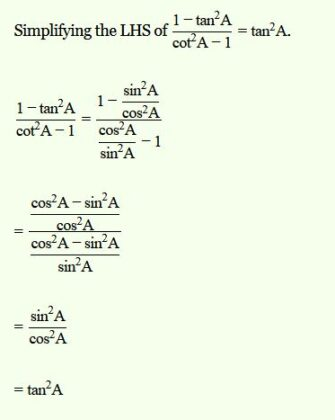28. A survey conducted on 20 households in a locality by a group of students resulted in the following frequency table for the number of family members in a household.
 Family size 1-3 3-5 5-7 7-9 9-11 Number of families 7 8 2 2 1
Solution

Here, modal class

and

Mode
Given mode value in question is correct.

#### Related News for you

Scroll to Top